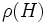# Fully invariant closure

This article defines a subgroup operator related to the subgroup property fully invariant subgroup. By subgroup operator is meant an operator that takes as input a subgroup of a group and outputs a subgroup of the same group.

## Contents

This article is about a definition in group theory that is standard among the group theory community (or sub-community that dabbles in such things) but is not very basic or common for people outside.
VIEW RELATED: Analogues of this | Variations of this | Opposites of this |
View a list of other standard non-basic definitions

## Definition

### Symbol-free definition

The fully invariant closure of a subgroup in a group can be defined in any of the following equivalent ways:

• As the intersection of all fully invariant subgroups containing the given subgroup
• As the subgroup generated by all endomorphs to the given subgroup
• As the set of all elements that can be written as products of finite length of elements from the subgroup and their endomorphic images

### Definition with symbols

The fully invariant closure of a subgroup$H$ in a group$G$, is defined in the following equivalent ways:

• As the intersection of all fully invariant subgroups of$G$ containing$H$
• As the subgroup generated by all$\rho(H)$ where$\rho \in \operatorname{End}(G)$